## Introduction

When converting spatial features from a geographic coordinate system (GCS) to a projected coordinate system (PCS) one or more spatial properties may be distorted in the transformation. These properties include area, shape, distance and direction .

Nicolas Tissot’s indicatrix (TI) is designed to quantify the amount of distortion in a map projection. The idea is to project a small circle (i.e. small enough so that the distortion remains relatively uniform across the circle’s extent) and to measure its distorted shape on the projected map.

For example, the following figure shows TIs across the continental US when presented in a Gnomonic projection centered at 100° West and 30° North.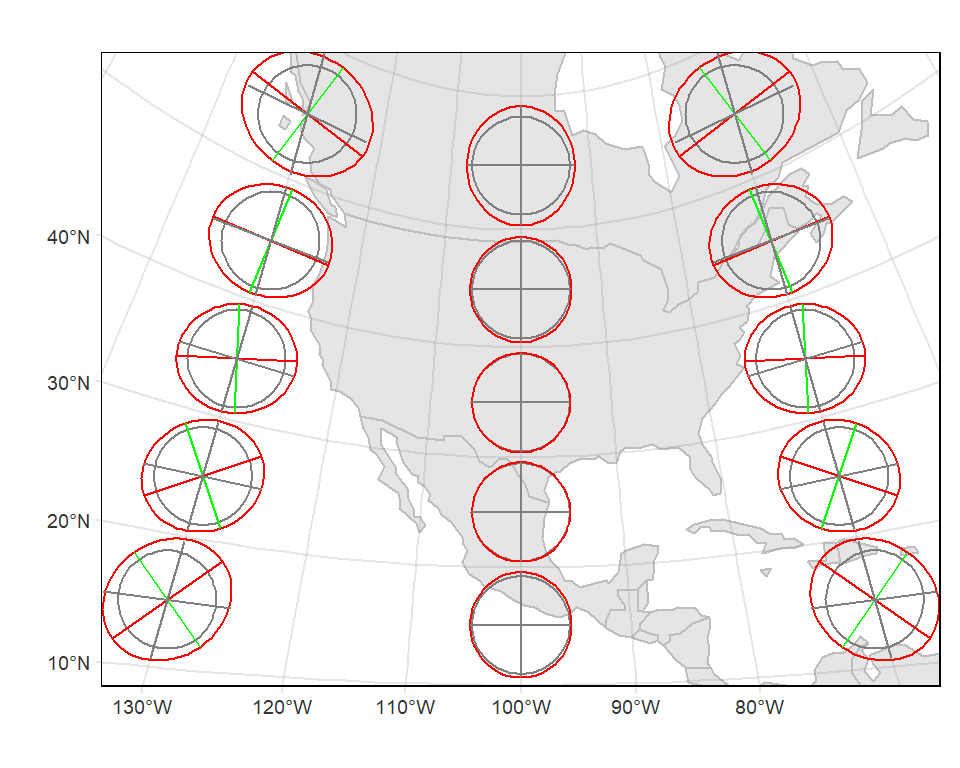Let’s explore a Tissot indicatrix (TI) in more detail at -69.5° West and 44.5° North (somewhere in central Maine, USA).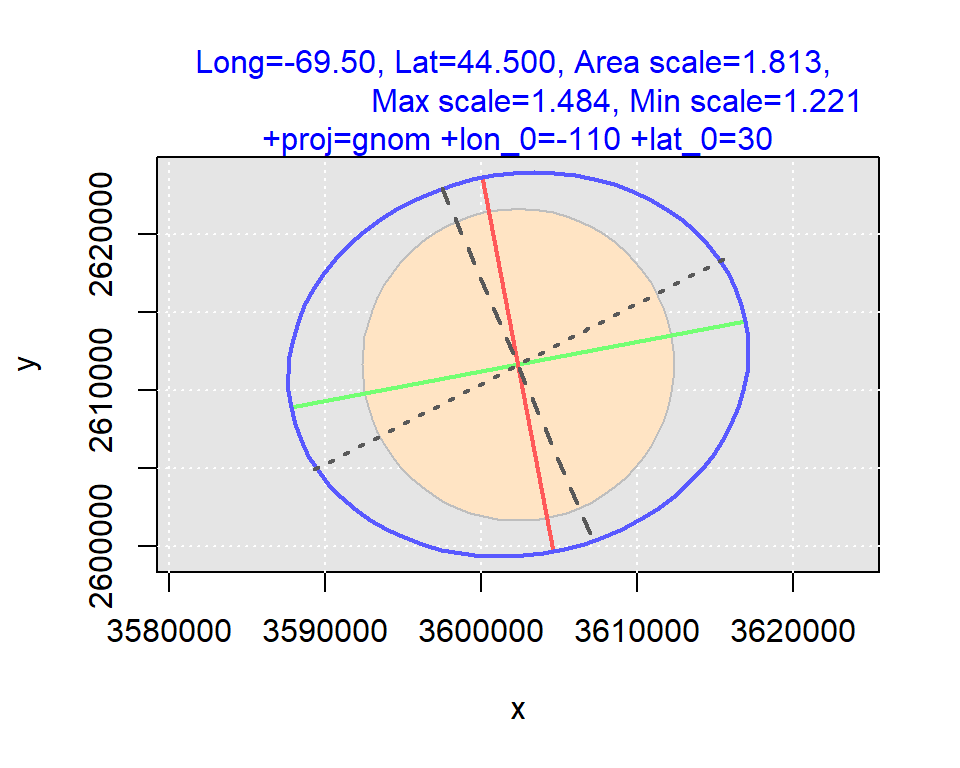The blue ellipse (the indicatrix) is the transformed circle in this particular projection (+proj=gnom +lon_0=-110 +lat_0=30). The green and red lines show the magnitude and direction of the ellipse’s major and minor axes respectively. If these axes are not equal (i.e. if the ellipse has a non-zero eccentricity), the projection is said not to be conformal at this location. These lines can also be used to assess scale distortion which can vary as a function of bearing as seen in this example. The green line shows the largest scale distortion and the red line shows the smallest scale distortion–these are sometimes referred to as the principal directions. In this working example, the principal directions are 1.484 and 1.221 respectively. A scale value of `1` indicates no distortion. A value less than `1` indicates a smaller-than-true scale and a value greater than `1` indicates a greater-than-true scale.

Not only can shape be distorted, but its area can be as well. The bisque colored circle at the center of the ellipse represents a base circle as represented in this gnomonic projection. It’s much smaller than the indicatrix. The indicatrix is 1.813 times greater than the base circle. In other words, an area will be exaggerated 1.813 times at this location given this gnomonic projection.

Other features of this indicatrix include The north-south dashed line which is aligned with the meridian and the east-west dotted line which is aligned with the parallel. These lines are used to assess if meridians and parallels intersect at right angles.

## Generating Tissot circles

A group of functions are available on the github website and can be sourced via:

``````library(sf)
library(rgdal)
library(tmap)

source("https://raw.githubusercontent.com/mgimond/tissot/master/Tissot_functions.r")``````

This tutorial will also make use of a few base layers used as a reference.

``````world <-  readRDS(gzcon(url("https://github.com/mgimond/tissot/raw/master/smpl_world.rds")))

### Defining a few projections

If you are not familiar with R’s coordinate system environment, you might want to skim through this web site. In this next chunk of code, we’ll create a few projection objects for use later in this tutorial.

``````# USA Lambert Conformal
proj.lcc    <- "+proj=lcc +lat_1=33 +lat_2=45 +lat_0=39 +lon_0=-96 +datum=NAD83"
# Equal area conic
proj.aea    <- "+proj=aea +lat_1=30 +lat_2=45 +lat_0=37.5 +lon_0=-96 +datum=NAD83"
# Equidistant conic
proj.eqdc   <- "+proj=eqdc +lat_0=37.5 +lon_0=-96 +lat_1=30 +lat_2=45 +datum=WGS84"
# Mercator
proj.merc   <- "+proj=merc +ellps=WGS84"
# Planar projection
proj.ortho1 <- "+proj=ortho +lon_0=-69 +lat_0=45 +ellps=WGS84"
proj.utm19N <- "+proj=utm +zone=19 +ellps=GRS80 +datum=NAD83"
# Equal Area Cylindrical projection
proj.cea    <- "+proj=cea +lon_0=0 +lat_ts=0 +x_0=0 +y_0=0 +ellps=WGS84"
# Plate Carree
proj.carree <- "+proj=eqc +lat_ts=0 +lat_0=0 +lon_0=0 +ellps=WGS84 +datum=WGS84"
# Azimuthal Equidistant
proj.aeqd   <- "+proj=aeqd +lat_0=45 +lon_0=-69 +datum=WGS84"
# Gnomonic
proj.gnom   <- "+proj=gnom +lon_0=-100 +lat_0=30 +ellps=WGS84" ``````

We’ll also define the input coordinate system used throughout this exercise. This CS will be used to define the lat/long values of the TI centers.

``proj.in <- "+proj=longlat +datum=WGS84"``

### Mercator projection indicatrix

We’ll start off by exploring a Mercator projection (a popular projection found on many mapping websites).

First, we’ll define point locations where we will want to evaluate projection distortions. We’ll automate the point creation process by gridding the point distribution.

``````lat <- seq(-80,80, by=20L)
lon <- seq(-170,170, by=34L)
coord <- as.matrix(expand.grid(lon,lat))``````

Next, we’ll run the `coord_check()` function (one of the functions sourced earlier in this tutorial) to remove any points that may fall outside of the Mercator’s practical extent.

``coord2 <- coord_check(coord, proj.out = proj.merc)``

Next, we’ll generate the indicatrix parameters for these points using the custom `ti()` function.

``i.lst <- apply(coord2,1, function(x) ti(coord = x, proj.out = proj.merc))``

Next, we’ll create `sf` objects from these indicatrix parameters.

``tsf <- tissot_sf(i.lst, proj.out = proj.merc)``

The output object `tsf` is a list consisting of the different indicatrix `sf` features. Each feature can be extracted from the list via its component name:

• `tsf\$base` The base circle as represented by the projected CS (polygon)
• `tsf\$ind` The indicatrix (polygon)
• `tsf\$maja` The semi-major axis (polyline)
• `tsf\$mina` The semi-minor axis (polyline)
• `tsf\$lam` The parallel (polyline)
• `tsf\$phi` The meridian (polyline)

Finally, we’ll plot the ellipses using the `tmap` package.

``````tm_shape(world, projection = CRS(proj.merc), bbox = tsf\$ind) +
tm_polygons(col = "grey80", border.col = "white") +
tm_shape(tsf\$base) + tm_borders(col="grey50") +
tm_shape(tsf\$ind)  + tm_borders(col="red") +
tm_shape(tsf\$mina) + tm_lines(col="red") +
tm_shape(tsf\$maja) + tm_lines(col="green") +
tm_shape(tsf\$lam)  + tm_lines(col="grey50") +
tm_shape(tsf\$phi)  + tm_lines(col="grey50") +
tm_graticules(alpha=0.2) ``````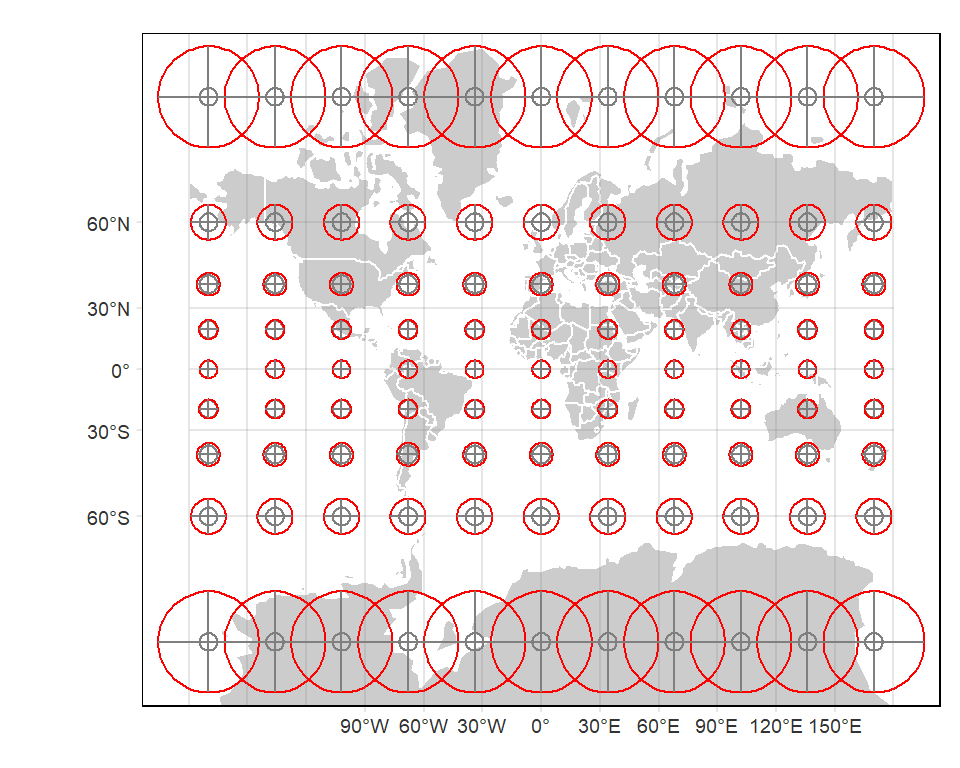The Mercator projection is conformal in that the shape of the circle remains a circle. Also, its lines of longitude and latitude remain at right angles across the entire map extent. Its area and scale, however, is heavily distorted as one approaches the poles.

A separate function, `TI_local()` is available that allows you to explore the indicatrix in more detail at a single point location. For example, to explore the Mercator distortion at a location in central Maine (USA), type:

``ti.maine <- local_TI(long = -69.5, lat = 44.5, proj.out = proj.merc)``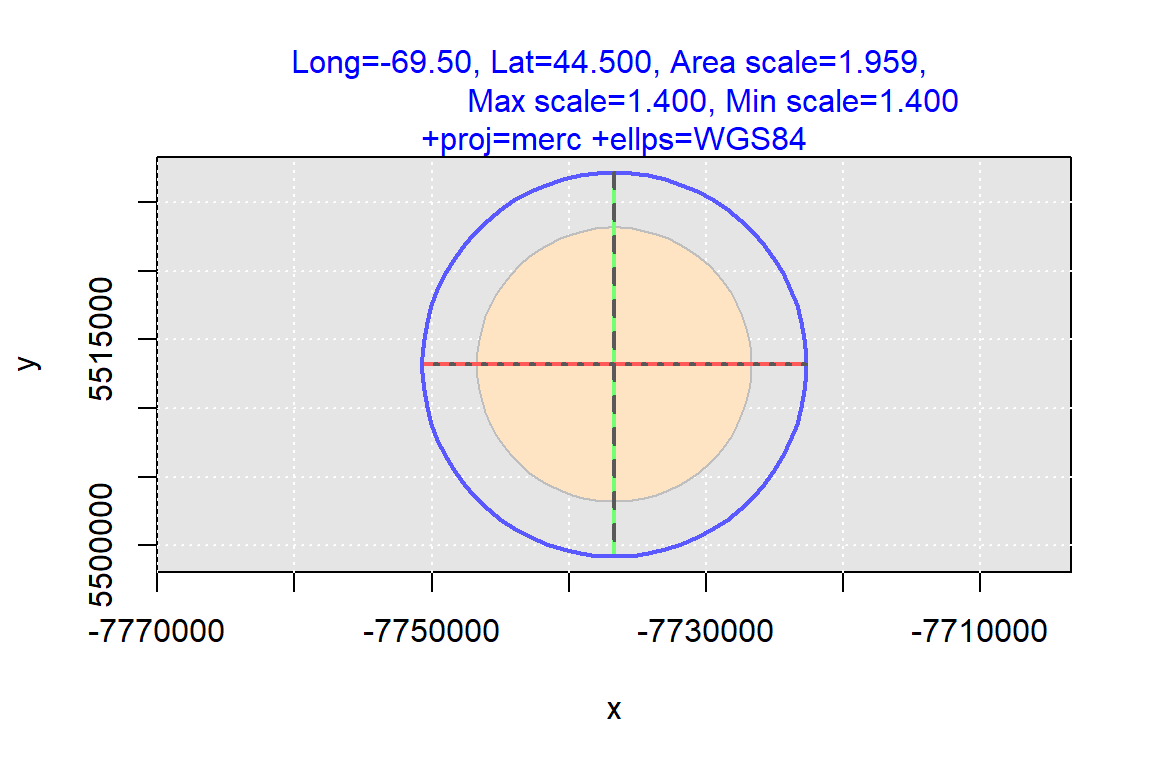You can extract indicatrix parameters from the `ti.maine` object generated from this function. For example, to extract the area scale, type:

``ti.maine\$scale.area``
`` 1.959244``

Likewise, to extract the principal direction scales, type:

``ti.maine\$max.scale``
`` 1.399736``
``ti.maine\$min.scale``
`` 1.399725``

### Custom function

Going forward, we will rerun many of the code chunks executed earlier in this exercise. To reduce code clutter going forward, we will create a custom function that will take as input projection type (`proj.out`) and tissot center locations.

``````ti.out <- function(proj.in = proj.in, proj.out, lat, lon, bmap = world,
plot = TRUE, base_fill = TRUE,
grt.lon = seq(-180,180,by=30), grt.lat = seq(-90,90,by=30)){
coord1 <- as.matrix(expand.grid(lon,lat))
coord2 <- coord_check(lonlat =coord1, proj.out = proj.out)
i.lst <- apply(coord2,1, function(x) ti(coord = x, proj.out = proj.out))
tsf <- tissot_sf(i.lst, proj.out = proj.out)

if(plot == TRUE){
# Re-project the world
bmap.prj <- st_transform(bmap, crs = proj.out, check = TRUE)

# Create graticule
grt <- st_graticule(crs = proj.in, lon = grt.lon, lat = grt.lat)

base.out <- if(base_fill) {
tm_polygons(col="grey80", border.col = "white")
} else {
tm_borders(col="grey50")
}

# Generate plot
tm_shape(bmap.prj, bbox = tsf\$ind) +
base.out +
tm_shape(tsf\$base) + tm_borders(col="grey50") +
tm_shape(tsf\$ind)  + tm_borders(col="red") +
tm_shape(tsf\$mina) + tm_lines(col="red") +
tm_shape(tsf\$maja) + tm_lines(col="green") +
tm_shape(tsf\$lam)  + tm_lines(col="grey50") +
tm_shape(tsf\$phi)  + tm_lines(col="grey50") +
tm_shape(grt) + tm_lines(col = "grey80")
} else{
return(tsf)
}
}``````

### Equal area cylindrical projection

Next, we’ll explore another cylindrical coordinate system that, unlike the Mercator projection, preserves area.

We’ll run the same chunks of code used in the last section, but we’ll replace all references to the output projected coordinate system with the `proj.cea` projection object.

``````# Define point coordinates for TI polygons
lat <- seq(-80, 80, by = 20L)
lon <- seq(-170, 170, by = 34L)

# Generate map
ti.out(proj.out = proj.cea, lat = lat, lon = lon)``````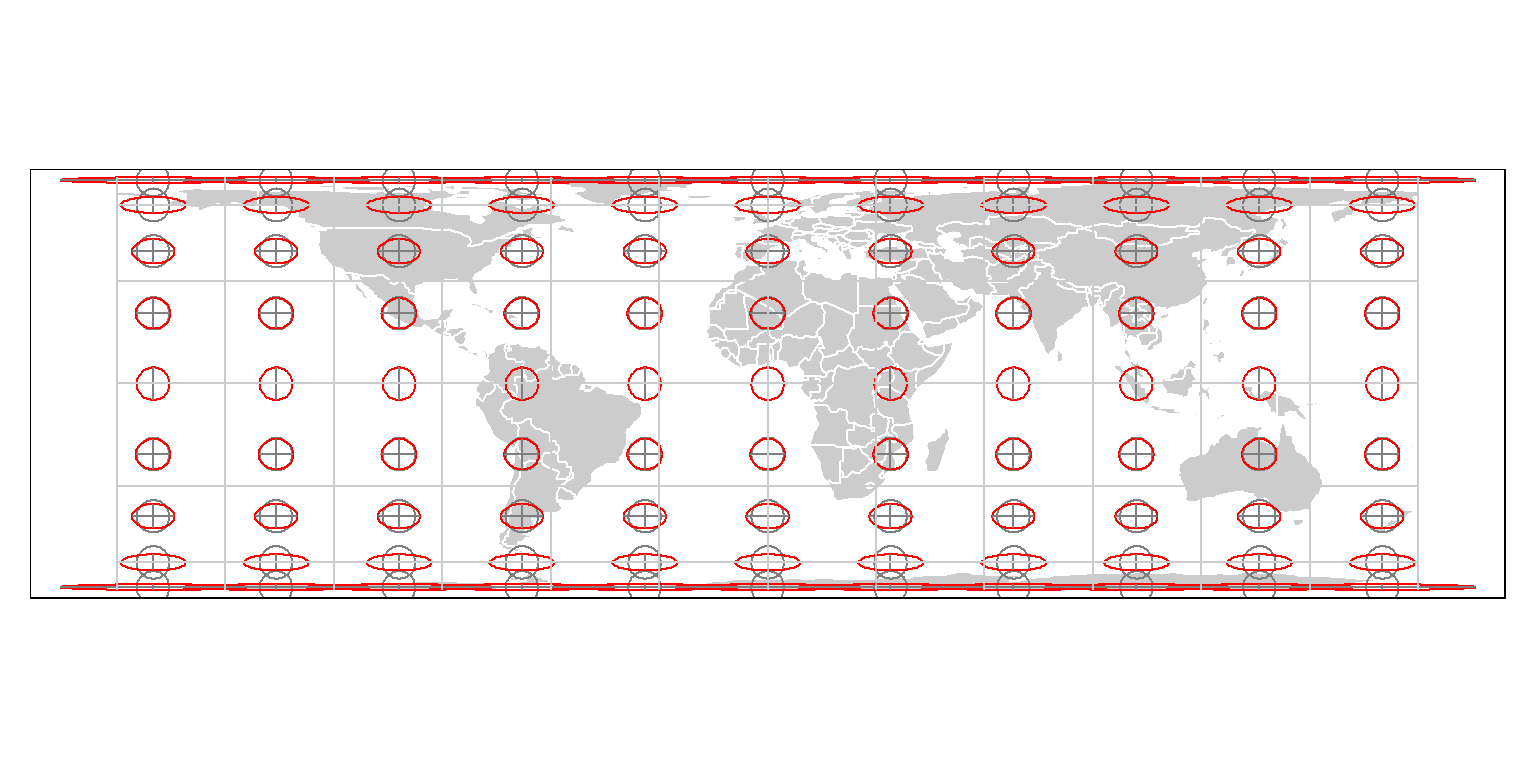Note the flattening of features as one progresses pole-ward. This compensates for the east-west stretching of features nearer the poles. Let’s check an indicatrix up close at the 44.5° latitude.

``ti.maine <- local_TI(long = -69.5, lat = 44.5, proj.out = proj.cea)``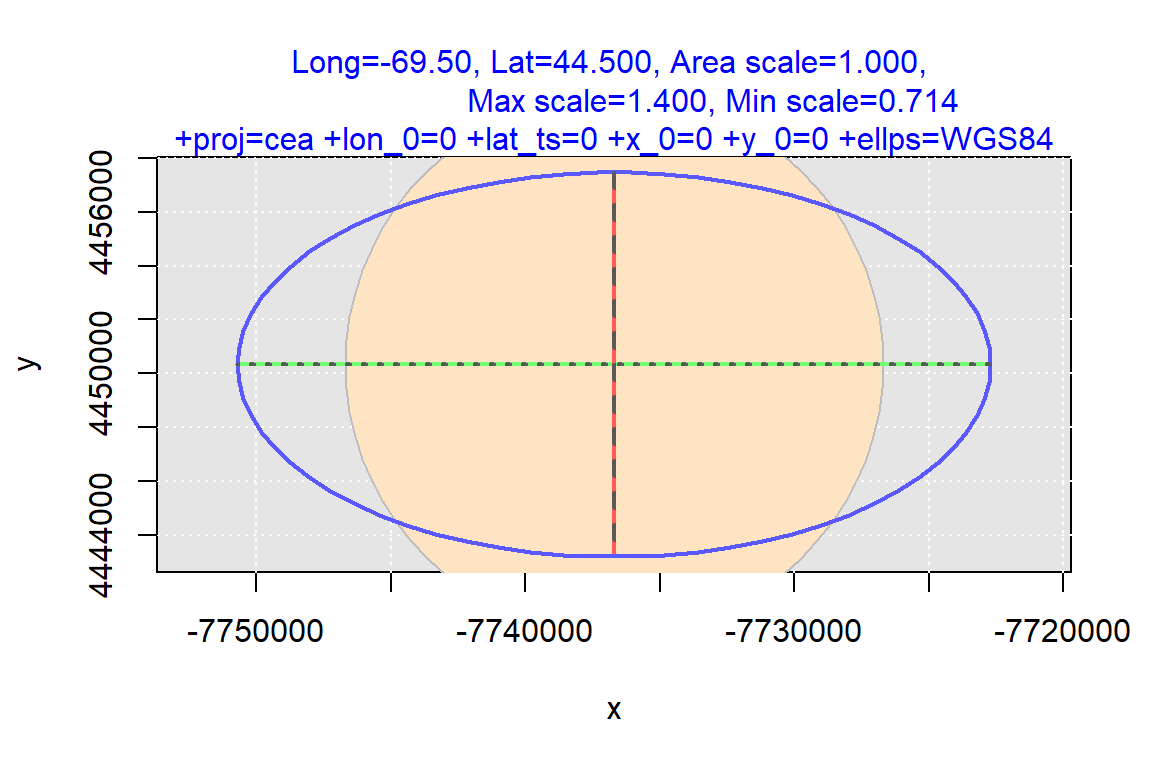The area is indeed preserved, but this comes at a cost. The projection is not conformal except near the equator (this is where the projection makes contact with the earth’s ellipsoid). At the 44.5° latitude, the east-west scale is increased by 1.4 and decreased by 0.7 along the north-south axis.

### Earth-from-space planar projection

Let’s explore another family of projections: an orthographic projection.

``````lat <- seq(-20, 80, by = 20L)
lon <- seq(-160, 40, by = 40L)
ti.out(proj.out = proj.ortho1, lat = lat, lon = lon)``````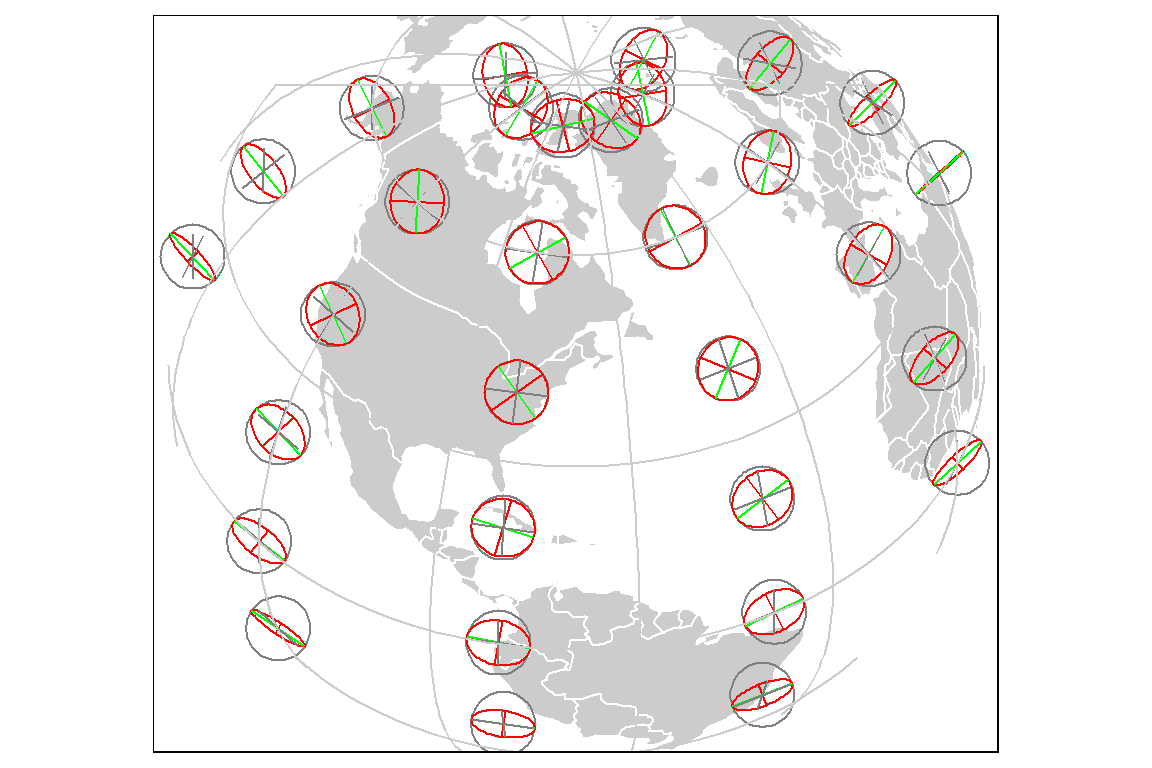This particular coordinate system has the center of the projection touching the earth’s surface. As such, you expect minimal distortion at the center (here the projection is centered at -69° longitude and 45° latitude).

``local_TI(long = -69, lat = 45, proj.out = proj.ortho1)``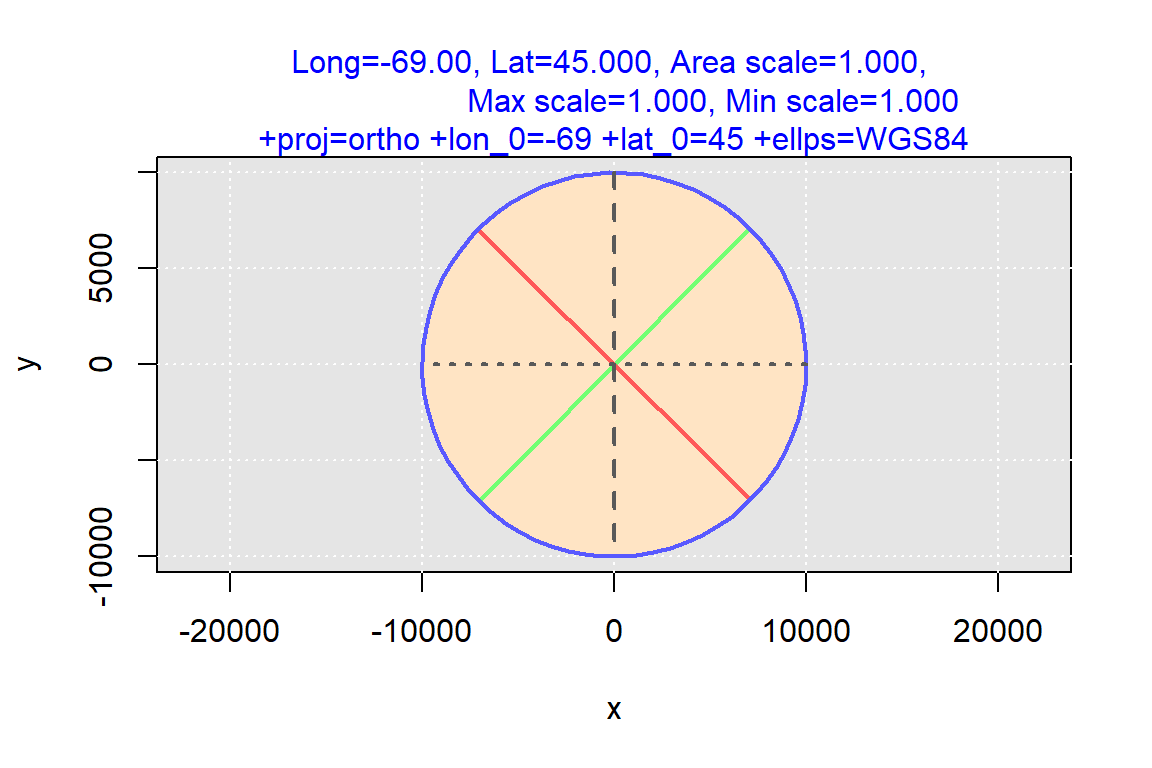Distortion in area and length increases as one moves away from the projection’s center as shown in the next TI centered at 0° longitude and 80° latitude.

``local_TI(long = 0, lat = 80, proj.out = proj.ortho1)``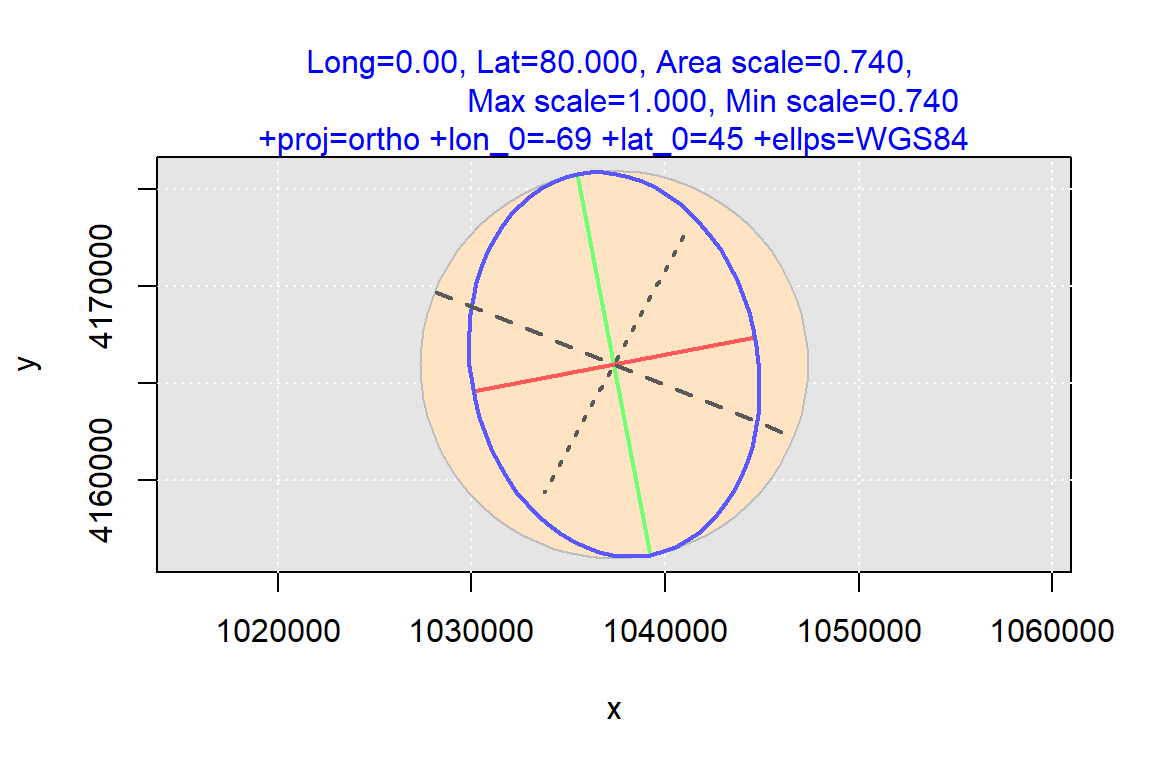### USA Contiguous Lambert Conformal Conic Projection

``````lat <- seq(20, 60, by = 20L)
lon <- seq(-130, -70, by = 30L)
ti.out(proj.out = proj.lcc, lat = lat, lon = lon, bmap = us)``````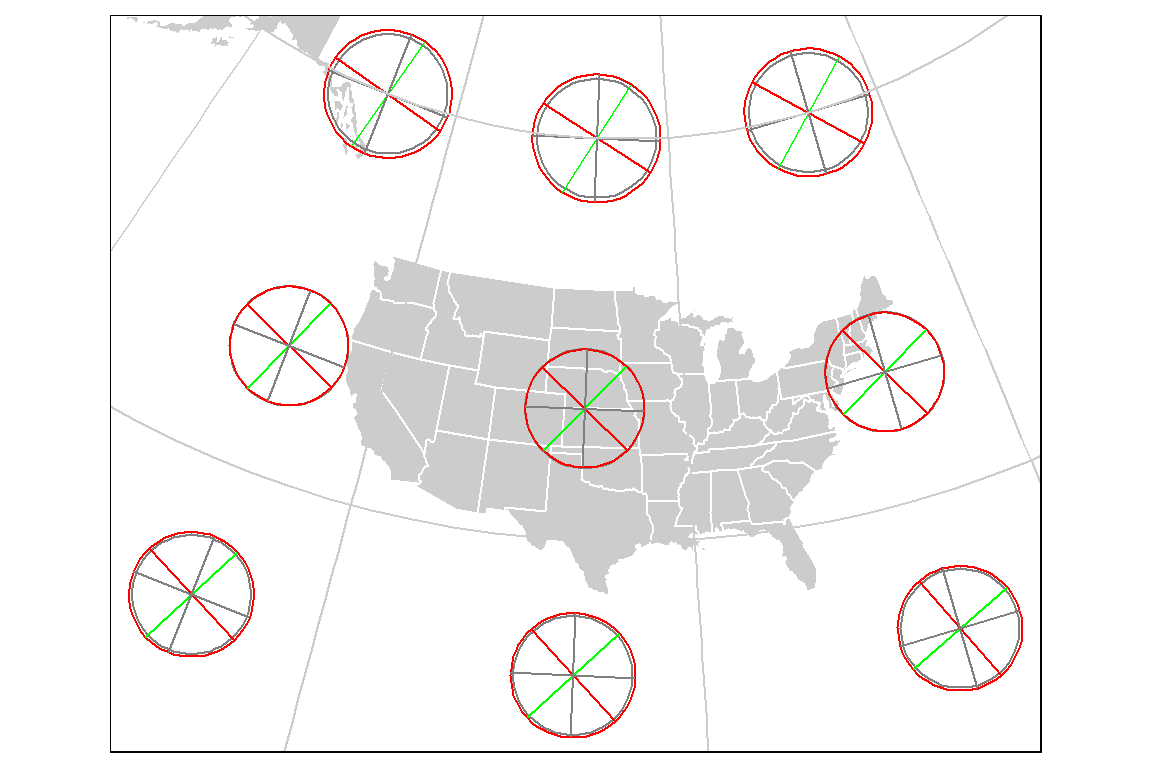Let’s explore one of the TI polygons in greater detail. We’ll zoom in on (60°N, 130°W).

``ti_lcc <- local_TI(long = -130, lat = 60, proj.out = proj.lcc)``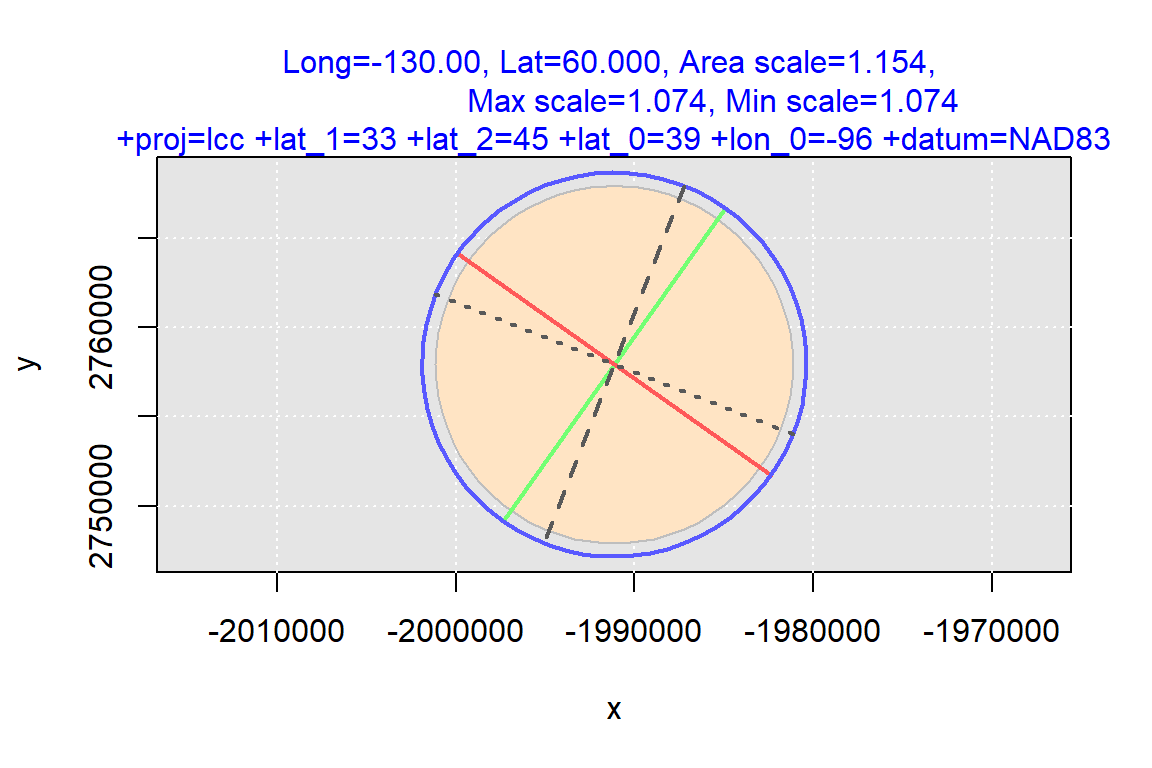The TI appears circular. We can quantify the distortion in shape by extracting the angle between the parallel and meridian lines at the TI’s center from the `ti_lcc` object (these are shown as the two dashed lines in the TI). A conformal projection is one that preserves angle, so we would expect a value of 90° between the two reference lines.

``ti_lcc[]  # Extract the intersecting angle``
``````intersection_angle
89.99917 ``````

This is very close to `0`. But it comes at a cost: distortions in area and length scales:

``ti_lcc\$scale.area``
`` 1.154365``
``ti_lcc\$max.scale``
`` 1.074422``
``ti_lcc\$min.scale``
`` 1.074405``

Note that for this projection to preserve shape, both principal directions most have the same length (as is the case in our working example with a scale of 1.074).

## Generating areal scale factor raster

We can generate a continuous (raster) surface of areal scale values. This can prove helpful in visualizing the distortion associated with the PCS across the whole map extent.

We’ll first create a custom function

``````library(raster)

r.out <- function(ll, ur, prj.out, plot = TRUE, grt = TRUE, bgmap = world,
nrows = 28, ncols = 32) {
ll.prj <- prj2(ll, proj.out = prj.out)
ur.prj <- prj2(ur, proj.out = prj.out)

# Define raster extent in the native PCS (note that the choice of extent and
# grid size may generate instabilities in the projected values)
ext.prj <- extent(matrix(c(ll.prj, ll.prj, ur.prj, ur.prj), nrow = 2))
r <- raster(ext.prj, nrows = nrows, ncols = ncols, crs = prj.out, vals = NA)

# Extract coordinate values in native PCS
coord.prj <- raster::coordinates(r)

# Now convert to lat/long values
# Note that we are reversing the transformation direction, we are going
# from projected to geographic.
coord.ll <- t(apply(coord.prj, 1,
FUN = function(x) prj2(x, proj.in = prj.out,
proj.out="+proj=longlat +datum=WGS84")))

# Compute Tissot area
i.lst <- apply(coord.ll, 1, function(x) ti_area(coord=x, proj.out = prj.out))
r[] <- round(i.lst,4)

# Reproject bgmap
bgmap.prj <- st_transform(bgmap, proj.out, check = TRUE)

# Map the raster
out <- tm_shape(r) +
tm_raster(palette = "Spectral", style="cont", midpoint = 1, title = "Scale") +
tm_shape(bgmap.prj) + tm_borders(col="grey20") +
tm_legend(outside = TRUE)

if (grt == TRUE) out <- out + tm_graticules()

if (plot == TRUE){
out
}else{
return(r)
}
}``````

### Orthographic projection

We’ll extend our analysis of the orthographic projection from the last section.

``````proj.out <- proj.ortho1
ll <- c(xmin = -90, ymin = 30)
ur <- c(xmax = -30, ymax = 60)

# Create raster map (remove default graticule which is problematic for this
# projection)
out1 <- r.out(ll, ur, proj.out, plot = TRUE, grt= FALSE)

# Create a custom graticule
# tm_graticule() introduces artifacts for this orthographic projection,
# so a separate graticule is generated for this projection
grt <- st_graticule(x = c(-155, 0, -5, 90), crs = proj.in)

# Plot the data
out1 + tm_shape(grt) + tm_lines(col = "grey80")``````